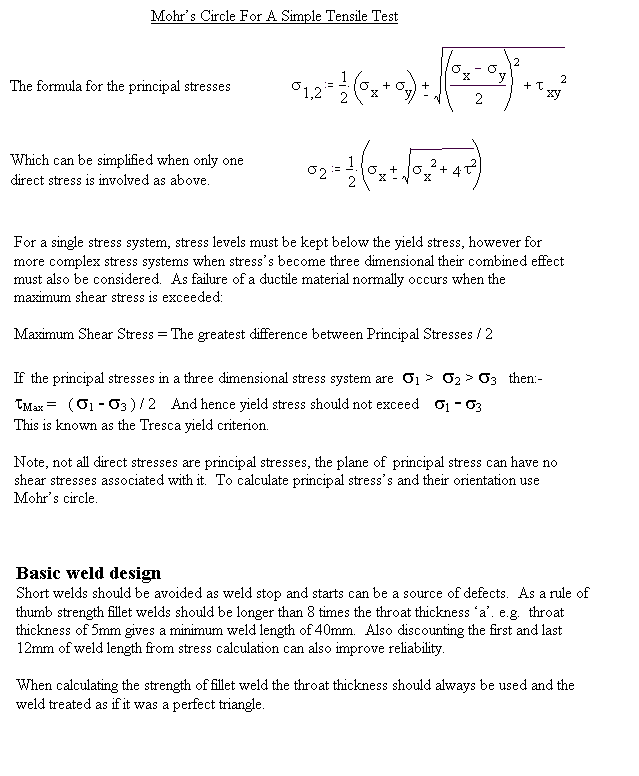## welding formula with Latex

• Post author:
• Post category:General

The formula for the principal stress: $latex \Omega _1,_2:=\frac{1}{2}\cdot(\Omega_x+\Omega_y)\pm \sqrt{(\frac{(\Omega_x-\Omega_y)}{2})^{2}+\tau_Xxy^{2}}$

This was an attempt to write a formula with Latex and Beautiful Math .  A lot of fun. I would like a project to learn more of this 🙂